## A mass of 100 g stretches a spring 5 cm. If the mass is set in motion from its equilibrium position with a downward velocity of 10 cm/s, and

Question

A mass of 100 g stretches a spring 5 cm. If the mass is set in motion from its equilibrium position with a downward velocity of 10 cm/s, and if there is no damping, determine the position u of the mass at any time t. When does the mass first return to its equilibrium position

in progress 0
5 months 2021-08-17T11:57:09+00:00 1 Answers 0 views 0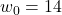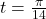Explanation:

Data

mass m= 100g

Length L= 5cm

we can use:

gm-kL= 0

divide both side by m

g –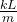=0

where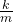=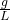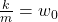^{2}

so now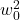=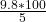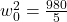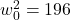square both side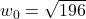We can apply:

u(t)=Acoswt +Bsinwt

u(t)=Acos14t +Bsin14t

u(0)=0  where A=0

therefore

u(0) = Bsin14t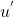(0) = 10 ⇒ 10=14B ⇒ B=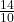B=so now u(t)=sin14t

so t will be:

t=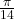t=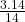t=0.22 seconds